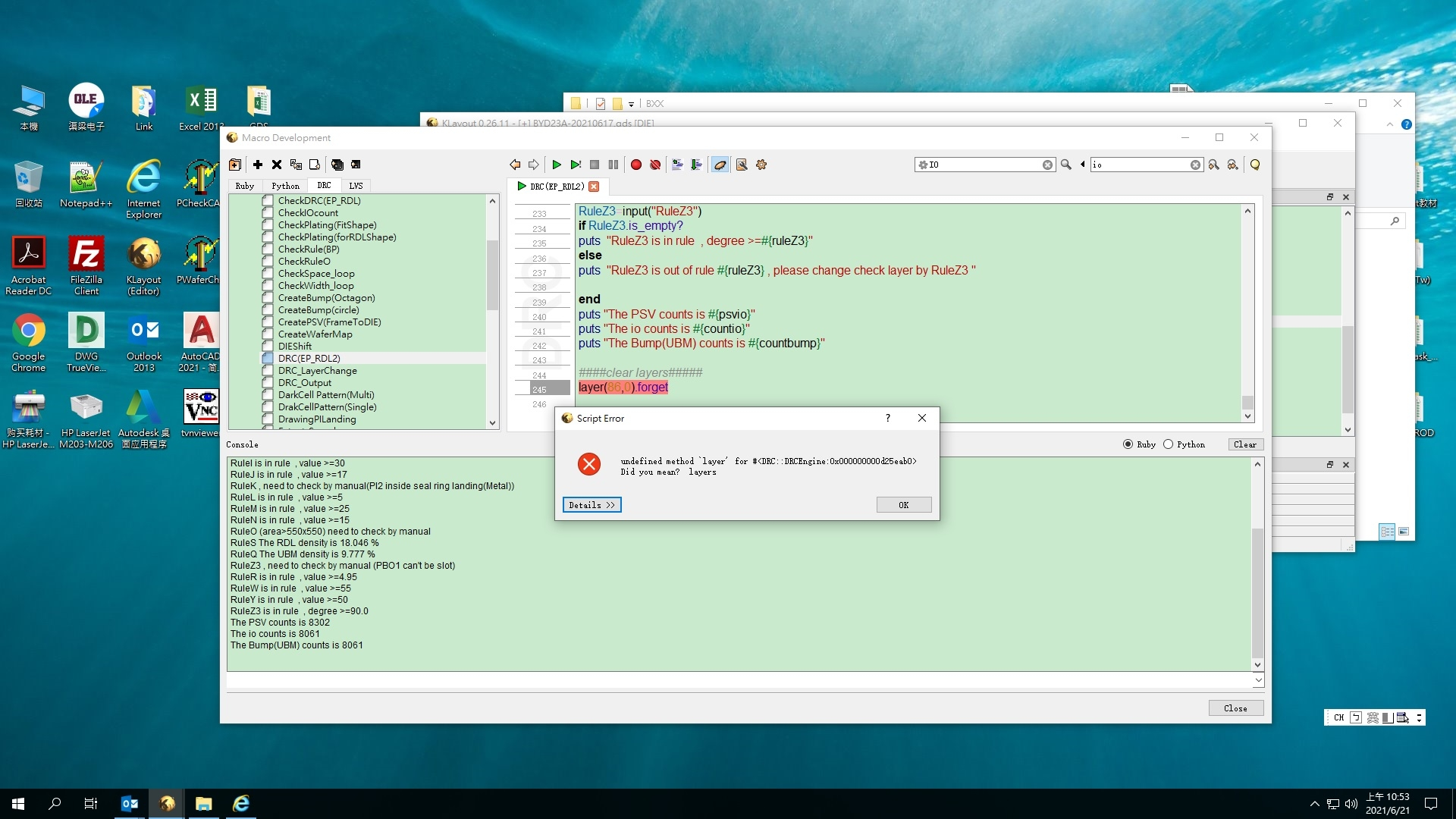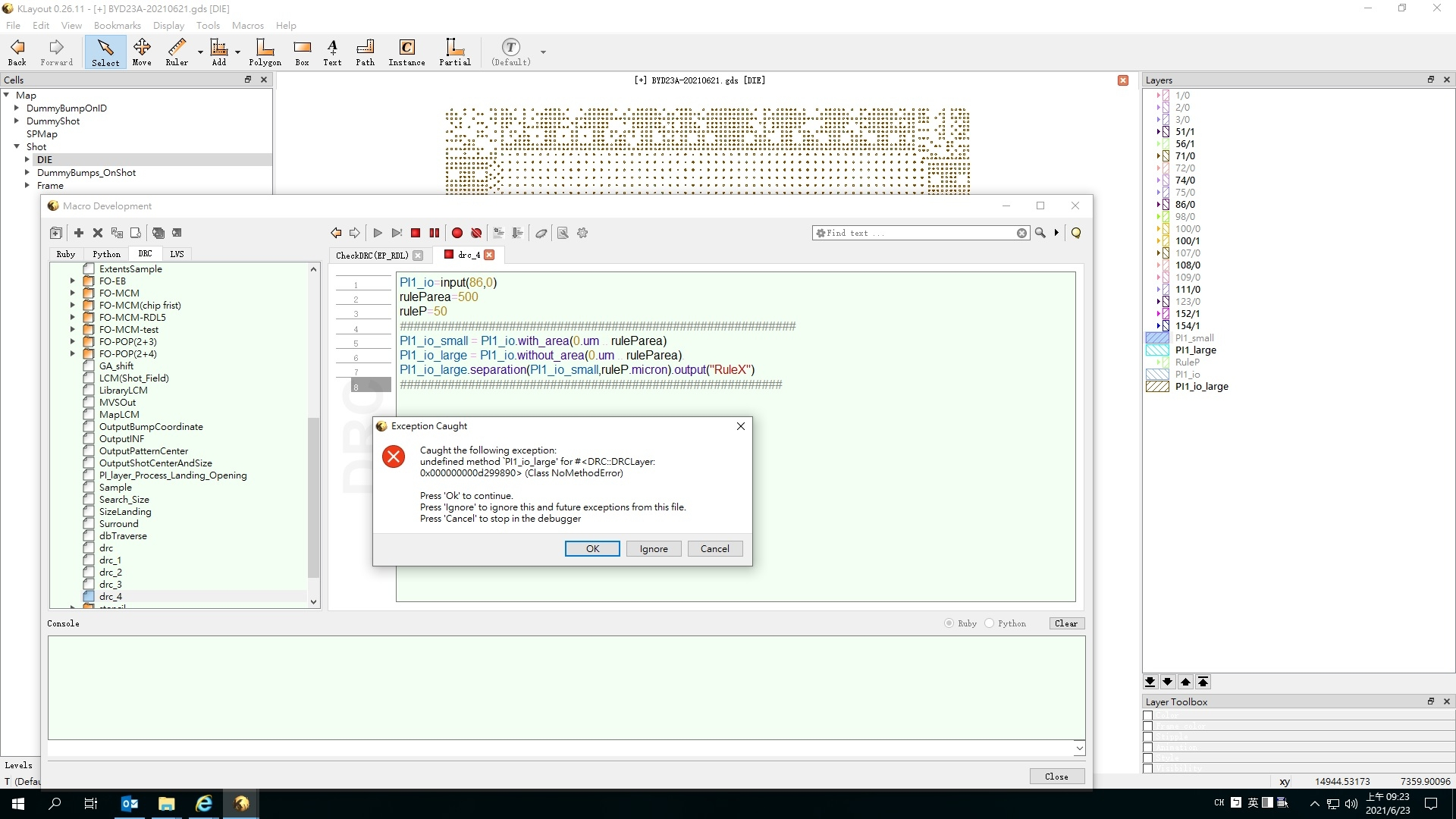# How to Clear the DRC template layer

``````PI1.inside(DIE).output(51,1000)
PI1_IO=input(51,1000)
PI1.not_inside(DIE).output(51,1001)
PI1_edge=input(51,1001)

PI2.inside(DIE).output(56,1000)
PI2_IO=input(56,1000)
PI2.not_inside(DIE).output(56,1001)
PI2_edge=input(56,1001)
###check RuleL ####
``````

as this DRC code , we have to make some template layers during DRC .
after rule check , how to remvoe /clear these templete layers (by DRC code).

I have try the way as below , but it is not work....

``````PI2_IO.not(PI2_IO).output(56,1000)
PI2_edge.not(PI2_edge).output(56,1001)
``````

• @jiunnweiyeh You do not need to generate output layers if you do not need the layer later.

``````PI1.inside(DIE).output(51,1000)
PI1_IO=input(51,1000)
``````

you simply say

``````PI1_IO = PI1.inside(DIE)
``````

to save some memory you can delete a layer once you do not need it anymore using "forget":

``````PI1_IO.forget
``````

Matthias

• Hi Matthias,
Thanks a lot for your help.

• Hi Matthias,
Is it possible to using this code?
(I want to remove layer 86,0)

``````####clear layers#####
layer(86,0).forget
``````• edited June 2021

No. "forget" only frees memory of a temporary layer.

If you want to clear a layer in a DRC script, you can write an empty layer to it:

``````polygons.output(86, 0)
``````

Matthias

• Hi Matthias,
please let ms explain more detail, as this code

``````PI1_io=input(86,0)
ruleParea=500
ruleP=50
PI1_io.with_area(0.um .. ruleParea).output("PI1_io")
PI1_io_small = input("PI1_io")

PI1_io_large = PI1_io.without_area(0.um .. ruleParea)
PI1_io_large = input("PI1_io_large")
PI1_io_large.separation(PI1_io_small,ruleP.micron).output("RuleP")
``````

this code is workable , out it will output 2 new layer , 1 is "PI1_io" , another 1 is "PI1_io_large"

when I change the code as below...

``````PI1_io=input(86,0)
ruleParea=500
ruleP=50
PI1_io_small = PI1_io.with_area(0.um .. ruleParea)
PI1_io_large = PI1_io.without_area(0.um .. ruleParea).
PI1_io_large.separation(PI1_io_small,ruleP.micron).output("RuleX")
``````

it is not workable , and output a error message .this is why I want to using the code 1 for DRC .
and , cause I will output addtion template layer , in this case , it is "PI1_io_large" and "PI1_io_small"
these layers will make some confuse in designer , it is why I want to remove or empty the both layers.
even I copy a empty layer as your code
polygons.output("PI1_io_small")
I still can't make the both layers been empty..
``````PI1_io_large = PI1_io.without_area(0.um .. ruleParea).
This will make the parse think there is a method following